# Average Velocity

Definition: Average Velocity is displacement over total time. Mathematically: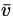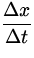=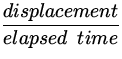(1)
Note:
• the overbar is frequently used to denote an average quantity
•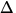t is always > 0 so the sign ofdepends only on the sign ofx .

Graphical interpretation of velocity: Consider 1-d motion from point P (with coordinates xi , ti ) to point Q (at xf , tf ). We can plot the trajectory on a graph (see Figure 2.1).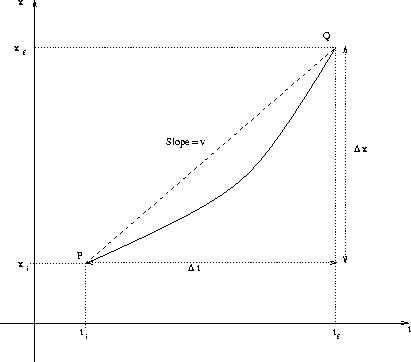Thenfrom Eq.(2.1) is just the slope of the line joining P and Q.

www-admin@theory.uwinnipeg.ca
10/9/1997